Test: Inverters - 1

# Test: Inverters - 1 - Electrical Engineering (EE)

Test Description

## 10 Questions MCQ Test GATE Electrical Engineering (EE) 2023 Mock Test Series - Test: Inverters - 1

Test: Inverters - 1 for Electrical Engineering (EE) 2023 is part of GATE Electrical Engineering (EE) 2023 Mock Test Series preparation. The Test: Inverters - 1 questions and answers have been prepared according to the Electrical Engineering (EE) exam syllabus.The Test: Inverters - 1 MCQs are made for Electrical Engineering (EE) 2023 Exam. Find important definitions, questions, notes, meanings, examples, exercises, MCQs and online tests for Test: Inverters - 1 below.
Solutions of Test: Inverters - 1 questions in English are available as part of our GATE Electrical Engineering (EE) 2023 Mock Test Series for Electrical Engineering (EE) & Test: Inverters - 1 solutions in Hindi for GATE Electrical Engineering (EE) 2023 Mock Test Series course. Download more important topics, notes, lectures and mock test series for Electrical Engineering (EE) Exam by signing up for free. Attempt Test: Inverters - 1 | 10 questions in 30 minutes | Mock test for Electrical Engineering (EE) preparation | Free important questions MCQ to study GATE Electrical Engineering (EE) 2023 Mock Test Series for Electrical Engineering (EE) Exam | Download free PDF with solutions
 1 Crore+ students have signed up on EduRev. Have you?
Test: Inverters - 1 - Question 1

### Assertion (A): For high power applications, inverters are used instead of transistors. Reason (R): For high power applications, inverter is operated in active region.

Detailed Solution for Test: Inverters - 1 - Question 1

In low-power electronic circuits oscillators are used for converting dc power into ac power. These oscillator use transistors for converting dc voltage into sinusoidal ac voltage. Since transistor is used in active region, therefore there is substantial loss of power which decreases efficiency. In high power applications inverters are used instead of transistors and the inverters operate in saturation region or cut-off region. Thus, assertion is true but reason is false.

Test: Inverters - 1 - Question 2

### Match List-l (Type of Inverter circuits) with List-ll (Characteristics) and select the correct answer using the codes given below the lists:  List-I  A. Voltage Source Inverter B. 120° Conduction Inverter C. Current Source Inverter D. 180°Conduction Inverter List-II 1. Suitable for a load of low leakage impedance 2. Each device turns on at regular interval of 60º 3. Suitable for multi machine control 4. Preferable for a delta connected load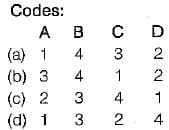Test: Inverters - 1 - Question 3

### Consider the following statements associated with CSI and VSI: 1. In CSI, commutation is load dependent process. 2. Thyristorised current source fed inverter has inherent four quadrant operation. 3. In VSl dynamic braking is applicable during ac line failure. 4. MOSFET and transistor are more suitable for CSl compared to VSI. Which of the statements given above is/are correct?

Detailed Solution for Test: Inverters - 1 - Question 3

• In CSI at light load, commutation time is considerably increased, which can restrict the highest frequency. Hence, commutation is load dependent process in CSI. Thus, statement-1 is correct.
• A VSl requires an additional line commutated converter for reverse power flow while it is hot so for a CSI i.e. a CSI has inherent four quadrant operation. Thus, statement-2 is correct.
• In VSl, dynamic braking is applicable during ac line failure. Thus, statement-3 is correct.
• MOSFET and transistor are more suitable for VSl not for CSI because in CSI large transient voltage is produced during commutation. Hence, statement-4 is not correct.

Test: Inverters - 1 - Question 4

A single-phase half bridge inverter has supply voltage of 200 V. For a load resistance of 10 Ω, the output power is equal to

Detailed Solution for Test: Inverters - 1 - Question 4

Output is a square wave with an amplitude of 100V.
RMS value of a square wave is equal to its peak value.
So, rms value of output voltage is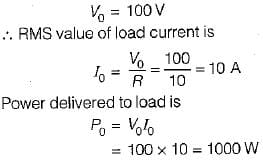Test: Inverters - 1 - Question 5

Assertion (A): The terminal voltage of a voltage source inverter remains substantially constant with variations in load.
Reason (R): Any short-circuit across the terminals of a voltage source inverter causes current to rise very fast.

Detailed Solution for Test: Inverters - 1 - Question 5

A voltage source inverter is one in which the d.c. source has small or negligible impedance. Due to low internal impedance, the terminal voltage of a VSI remains substantially constant with variations in load. Reason is also a correct -statement because due to low time constant of internal impedance any short-circuit across the terminals of a VSI causes current to rise very fast. Hence, both assertion and reason are true but reason is not the correct explanation of assertion.

Test: Inverters - 1 - Question 6

The single-phase half-bridge inverter has a resistive load of 10Ω and the centre-tap dc input voltage is 96 V. The fundamental power consumed by the load is

Detailed Solution for Test: Inverters - 1 - Question 6

The nth harmonic-component of output voltage is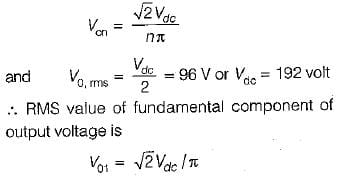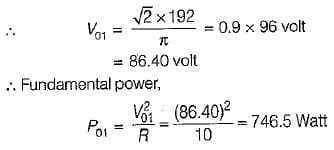Test: Inverters - 1 - Question 7

A single-phase half-bridge inverter has a resistive load of R = 3 Ω and the dc input voltage Vdc = 24 volts. The harmonic factor of the lowest order harmonic would be equal to

Detailed Solution for Test: Inverters - 1 - Question 7

The lowest harmonic is third harmonic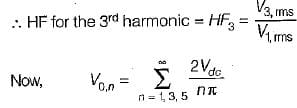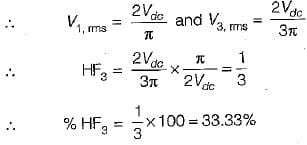Test: Inverters - 1 - Question 8

A single-phase transistorized bridge inverter has a resistive load of R = 3 Ω and the dc input voltage of 48 volts. The total harmonic distortion is approximately equal to

Detailed Solution for Test: Inverters - 1 - Question 8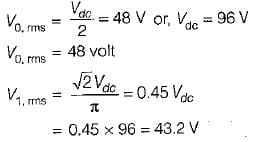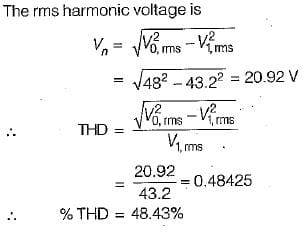Test: Inverters - 1 - Question 9

A full-bridge bipolar PWM inverter is fed from a 240 V battery and is driving an RL load. The fundamental output voltage for a modulation index of 0.8 is

Detailed Solution for Test: Inverters - 1 - Question 9

The fundamental output voltage for a modulation index of M is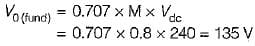Test: Inverters - 1 - Question 10

A current source inverter can be

Detailed Solution for Test: Inverters - 1 - Question 10

A CSl can be either load commutated or force commutated.

## GATE Electrical Engineering (EE) 2023 Mock Test Series

22 docs|284 tests
Information about Test: Inverters - 1 Page
In this test you can find the Exam questions for Test: Inverters - 1 solved & explained in the simplest way possible. Besides giving Questions and answers for Test: Inverters - 1, EduRev gives you an ample number of Online tests for practice

## GATE Electrical Engineering (EE) 2023 Mock Test Series

22 docs|284 tests(Scan QR code)